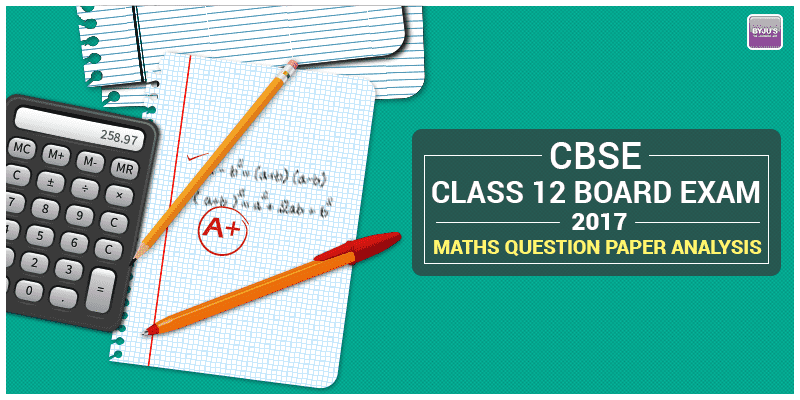# CBSE Class 12 Board Exam 2017: Maths Question Paper AnalysisCBSE class 12 is the Central Board Of Secondary education. The examination is held on the 9 March 2017 and this examination will continue until the end of the next month, i.e., 29 April 2017. The CBSE Class 12 Maths Board Exam 2017 is conducted on 20 March 2017.

From the survey, it is found that the total number of students appeared for the examination of CBSE class 12 are about 10,65,179 students. This year the number is increased, and the total number of students appearing for the examination class 12 2017 is about 10,98,420 students. From this survey, it has found that about 10677 students are increased this year. The mathematics examination is held on 20 March 2017, and the accountancy examination is held on 29 March 2017.

#### Here is the overall analysis of the maths question paper class 12 of CBSE:

After taking the CBSE Class 12 Maths examination, the different opinions among the students about the question paper. Some students say the paper was a bit lengthy, and some others say that this year question paper is a bit easy when compared to the previous year question paper. Social media is the biggest and best platform to give opinions, and most of the student’s opinion is that Maths is the toughest subject. Students say that they have attended about 80 to 90% of the questions in the mathematics question paper.

##### A) The difficulty level in the question paper:

First of all, the mathematics question is of three sets and most of the questions in the three are somewhat same. The question paper is much easier when compared to the last year or the previous year question paper. This year the question paper is not lengthy. In these three sections, only the two or three questions are different in some sections.

##### B) Average student score:

As most of the questions are from the textbook and the questions in all the three sets are almost same, so there are around 80 to 90% chances that an average student can attempt. So based on this analysis, we can say that the question is quite easy, and an average student can get a good score.

##### C) The noticeable changes in the question paper:

There are small changes in the mathematics question paper, but this is based on the weightage. The six marks compulsory question for the application of the derivatives is easy, but this is done only in one set, and in the remaining two sets the complete questions are from the textbook, so there is such difficulty in the chances part.

##### D) The Best thing about the question paper:

The overall best thing about the question paper is, this year the question paper is quite easy, and students can score more in the mathematics part this year. Most of the students say that the mathematics question paper is so hard, but, from this year, students get the confidence that they can crack the mathematics question paper easily.

##### E) Overall analysis of the mathematics question paper:

The only problem students faced this year is the question paper was lengthy, but they also posted on the twitter, and the Facebook is that the question is tricky, but it is easy so with this we can say the question is easy for the students who worked hard for them it is quite easy and these candidates can score the full score. Even the average students can also score more in this year examination.